## Friday, May 26, 2017

### Public Service Announcement

I am sad to say that it's been many a day since I have written a post here.  But now that my semester is over, I have finally gotten back to writing.  Some day, I'll have better time management skills and be able to write in this blog as often as I want.  But until that day comes, most of my writing will be done during seasons when class is not in session.

On another note, I have an important announcement.

From now on, I will continue writing my blog on my own website: http://mwilson1.github.io
So, to all of my fans across the world, you'll have to go there from now on to keep up with all of my astronomy thoughts.  See ya there!

## Friday, August 5, 2016

Now that you understand why a proton or electron from the Sun that bombards the Earth's magnetic field lines undergoes cyclotron motion, let's make things slightly more complicated.  Now, the magnetic fields that we'll deal with have a gradient.  Simply put, this means that the magnetic field line is stronger on one end than on the other sections of the magnetic field line.  In mathematical terms, the magnetic field vector $$\textbf{B}$$ is no longer constant with respect to position; thanks to the gradient, changes in $$\textbf{B}$$ only depend on location.  (For now, this vector is only a function of position--it remains constant over time.)   Just to clarify, I'll add that the Lorentz force--discussed in the previous post--applied by the $$\textbf{B}$$ field onto the particle is dependent on the direction, or angle with respect to the $$\textbf{B}$$ field, of the particle's velocity, but this force is not dependent on the location of the particle while it is inside of that magnetic field as long as that magnetic field has no gradient.
Pheww!! ... tough explanation ... it can be hard sometimes to explain the concept of vectors in contrast to vectors that are functions of position to people that might not utilize vector mathematics in their everyday lives.

The simulation I am about to show has a proton traveling in the presence of a magnetic field with a gradient.  For the sake of laziness, I'll just call this magnetic field a Gradient-B field ( $$\nabla B$$ ) from hereon.  It is important to note that because we are now dealing with $$\nabla B$$ field--a magnetic field where its strength changes depending on your location within the field--the Lorentz force applied to the proton will change as the proton travels from one location to another within this field.  When the proton enters a $$\nabla B$$ field, it drifts in a direction other than the direction of the magnetic field vector $$\textbf{B}$$.  In my previous post, the particle only drifted parallel to $$\textbf{B}$$ but as it drifted in that direction it also traveled in a circle--hence cyclotron motion.

The average velocity due to the $$\nabla B$$ drift is described as
$v_a = \frac{\mu}{q} \frac{ \textbf{B}\times \nabla_{\perp}B }{B^2}$
where mu $$\mu$$ is
$\mu = \frac{m v_{\perp}^2}{2B}$
and $$\nabla_{\perp}B$$ is the strength of the $$\nabla B$$  field in the direction perpendicular to the $$\textbf{B}$$ field.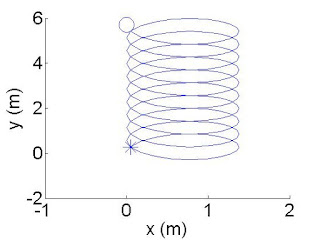Top View Star is the beginning and circle is end of simulation.
For this simulation, I used the following equation to describe the motion of a proton:
\begin{equation} \textbf{r}(t)-\textbf{r}(0) = \frac{v_{\perp}}{\Omega_c}[1 - cos(\Omega_c t)]\hat{\textbf{e}}_x + \frac{v_{\perp}}{\Omega_c}sin(\Omega_c t)\hat{\textbf{e}}_y + v_{\|}t\hat{\textbf{e}}_z + \frac{\mu}{q} \frac{ \textbf{B}\times \nabla_{\perp}B }{B^2}t
\end{equation}
however!! it is important to know that the initial velocity I set for my proton was $$v_{\perp} = \textbf{v}(0) = 4000$$ $$\hat{\textbf{e}}_y$$ m/s which means that the velocity of the proton when $$0$$ seconds has gone by is 4,000 meters per second only in the +y-direction.  I will also note that I set the $$\textbf{B}$$ field to point in the +z-direction but the $$\nabla B$$ vector is pointed in the +x-direction.  These details are important for understanding why the particle traveled the path that is shown in the video.

Because of the mathematics, I knew how the proton should have behaved but I needed to confirm my hypothesis with the video.
Here are some questions I asked myself when I first saw the video my code created:

Did the proton really undergo cyclotron motion?
Yes.  That's why it travels in that loopty-loop fashion, as seen in the "Top View" image.

Why did the proton drift in the +y-direction?
This is because the velocity drift due to $$\nabla B$$ is in that direction.  This is shown in the $$v_a$$ equation because of the cross product $$\textbf{B}\times \nabla_{\perp}B$$.  In terms of the directions, this cross product adheres to the right hand rule: $$\hat{\textbf{e}}_z \times \hat{\textbf{e}}_x = \hat{\textbf{e}}_y$$ , which is the +y-direction.

Why didn't the proton ever move in the $$\pm$$z-direction?
This is because my initial condition for velocity $$\textbf{v}(0) = 4000$$ $$\hat{ \textbf{e}}_y$$ m/s is not in the $$\pm$$z-direction nor does the Lorentz force $$\textbf{F} = q(\textbf{v} \times \textbf{B})$$ ever at any point in time push the proton in the $$\pm$$z-direction.

Why does the proton maneuver in both x and y directions if the initial condition of the proton started it off going in only the +y-direction?
This is because the Lorentz force accelerates the proton into +x-direction at the beginning ( $$\textbf{v} \times \textbf{B} == \hat{y} \times \hat{z} = \hat{x}$$ ) and as time goes on the Lorentz force continues to accelerate the particle in different directions until eventually the particle has gone a circular path.

Hopefully, I answered all of the questions that may or may not have already popped into your head when watching my code run.  I think that this is enough fun for today.  Next time, we'll see what flabbergasting events transpire in the simulation when the initial velocity changes.

## Friday, July 8, 2016

### Space Physics: Cyclotron Motion

I'll discuss a basic topic in Space Physics today that is certainly not a basic idea for the average person outside of science to understand.  However! that should not matter nor should it intimidate you!  Any person (such as myself) that attempts to teach ANY scientific concept should be capable of explaining their science in such a way that even children with little scientific background can understand the concept.(Credit: spaceweather.com)
The basic topic is the behavior of a proton as it approaches the Earth's magnetic field.  Remember, an atom--those boxes you see on a periodic table--can consist of a positively charged proton and a negatively charged electron.  Because I am discussing a topic in the field of space physics, I will also remind you that the Earth's magnetic field is always being hit by protons and electrons from the Sun.

Today, I will describe one of the ways a proton behaves when encountering an unchanging magnetic field line.

When a proton approaches a magnetic field line, the proton spirals around a magnetic field line and continues in the direction of that line.  This is known as cyclotron motion.Top View
The video illustrates a simulation in which a magnetic field line is aligned with the z-axis and is pointing upward in the +z direction (also denoted as the $$+\hat{\textbf{e}}_z$$ direction).  This is why the proton travels in the +z direction over time while moving in a circle.  Mathematically, the +z direction of the particle's motion is shown via the $$v_{\|}t\hat{\textbf{e}}_z$$ term at the end of my cyclotron motion derivation.

The image to the right provides a top view of the simulation.  This perspective allowed me to verify if the proton truly has a circular trajectory in 2D space.  It is easy to see (thanks to the numbers on the axes) that the proton traveled 0.23 meters in the +y direction as well as the -y direction.  The trajectory looks visually like an ellipse (ugghhhh, thanks MATLAB...) but after looking at the numbers, I found that the proton truly did travel 0.23 meters from the center of the circle in all directions.  The radius of this circular cyclotron motion is known as the Larmor orbital radius:
$r_L = \Big| \frac{v_{\perp}}{\Omega} \Big| = \Big| \frac{mv_{\perp}}{qB} \Big|$
where $$\Omega$$ is the frequency of the orbit, m is the mass of the particle (in this case a proton), $$v_{\perp}$$ is the component of velocity of the particle that goes in a direction that is perpendicular to the magnetic field B, and q is the charge of that particle (in this case +1.602$$\times$$10$$^{-19}$$ Coulombs).

Now, let's see how the electron behaves in the presence of a magnetic field line.  Notice anything different?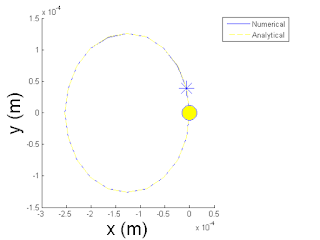Top View
Hmmmm... Interesante.  At first glance, it seems to have done the same thing as the proton.  One difference seen is that the electron's cyclotron motion is counterclockwise while the proton's motion is clockwise.  Another distinction is noticed when looking at the simulation from the top view.  The Larmor radius is now 1.26$$\times$$ 10$$^{-4}$$ meters.

Mathematically speaking, the reason why the electron's Larmor radius is shorter than the proton's Larmor radius is because the mass of an electron is about 1800 times less than the mass of a proton, and because the $$r_L$$ is directly proportional to the m, the super tiny electron mass makes its Larmor radius super tiny as well.

The difference in clockwise vs counterclockwise motion between proton and electron is due to the Lorentz force:
$\vec{F} = q(\vec{E} + \vec{v} \times \vec{B} )$
where $$\vec{E}$$ is the electric field.  Basically, this equation means that whenever a charged particle is in the presence of an electric or magnetic field, a force will be applied to that particle which will cause it to either to accelerate or change directions.  The arrows on top of the variables indicate that they are vectors, which means that the electric field strength, the particle's velocity, and the magnetic field strength are all pointed in some direction.  In my simulation, I have not included an electric field.  Therefore, in this case, $$\vec{E}=0$$ and $$\vec{F} = q ( \vec{v} \times \vec{B} )$$.  Between the proton and electron simulations I did not change the velocity nor the magnetic field.  The only thing that was different is the charge q.  Since protons and electrons have opposite charge, the magnetic field's force $$\vec{F}$$ was pushing them in opposite directions!  Before clicking on my previous videos, you could have predicted if the motion was going to be clockwise or counterclockwise as long as you would have used the right hand rule for the cross product $$\vec{v} \times \vec{B}$$.

Later, I will discuss other behaviors that particles from the Sun exhibit when they encounter the Earth's magnetic field.

Deriving Position from Velocity of Pure Cyclotron Motion
\begin{equation} \textbf{v}(t) = v_{\perp}sin(\Omega_c t)\hat{\textbf{e}}_x + v_{\perp}cos(\Omega_c t)\hat{\textbf{e}}_y + v_{\|}\hat{\textbf{e}}_z
\end{equation}
$\int_{t=0}^{t} \textbf{v}(t) dt = \int_{t=0}^{t} \big( v_{\perp}sin(\Omega_c t)\hat{\textbf{e}}_x + v_{\perp}cos(\Omega_c t)\hat{\textbf{e}}_y + v_{\|}\hat{\textbf{e}}_z \big)dt$
$\textbf{r}(t)-\textbf{r}(0) = -\frac{v_{\perp}}{\Omega_c}[cos(\Omega_c t) - 1]\hat{\textbf{e}}_x + \frac{v_{\perp}}{\Omega_c}[sin(\Omega_c t)- 0]\hat{\textbf{e}}_y + v_{\|}[t - 0]\hat{\textbf{e}}_z$
\begin{equation}\label{Eq2: Analy path} \textbf{r}(t)-\textbf{r}(0) = \frac{v_{\perp}}{\Omega_c}[1 - cos(\Omega_c t)]\hat{\textbf{e}}_x + \frac{v_{\perp}}{\Omega_c}sin(\Omega_c t)\hat{\textbf{e}}_y + v_{\|}t\hat{\textbf{e}}_z
\end{equation}

## Saturday, January 2, 2016

### Long Time, No See

Wow! it feels good to be back.

Many of you know, have seen, and have heard about the grand schemes I have been up to in the past few months, which has kept me too busy to write more blog posts here.  I'll take a moment to get you all up to speed on the big moves I have been making.

Some of you have seen the inception of my Research Website and the drastic changes I have made to it since I started writing the HTML/CSS/JavaScript code for that in September.  I even have a cool background image for the site now!  Look familiar?
Seeing this website come to fruition is like a small dream come true for me.  This started as a simple idea and at the start I only knew a couple HTML commands (< br > for break and < a > for links).  The website is still a work in progress but I love how it's turning out.Another endeavor (that only my research advisors probably noticed) is the amount of money I have racked up this year for research--as seen on my CV.  Receiving that much   \$ for research funding as an undergraduate, within one year's time, required me to devote a LOT of time to writing about my research.  (And my CV doesn't even include the generous stipend I received from my summer internship! )

Needless to say, all of that writing about my research took a lot of time away from my academic studies during this past fall semester.  The writing combined with the actual obligations of conducting the research certainly made this semester much more difficult.   However, I am happy to inform you that I persevered.  I didn't get a 4.0 GPA this semester, but I am still happy with the fruits of my labor.  Excellent grades, great progress in research, and an abundance of research funding came out of my hard work this summer and semester.

The McNair Scholars Program, formally known as the Post-Baccalaureate Achievement Program, also kept me busy.  Much of my extra-curricular writing this year was for the McNair Program.  Additionally, because of my completed McNair research project I had to present my research at the FIU McNair conference.Me delivering my talk.  For those of you who've seen my website, the photo should look familiar.
Hanging out with other McNair students that completed their research projects was a fun experience too.

We had a great time together.  The conference itself was mediocre though.  (With the AAS Meeting being my first science conference, I admit that I'm probably spoiled when it comes to this stuff.)

In this post, I have given you a brief synopsis for a few of my most time consuming (although fun) extra-curricular activities for this past semester.  However, I didn't even tell you about the hard work I put into my NSF Graduate Research Fellowship application.  Nor did I discuss the essays I have written this semester for graduate school applications.  But most importantly, YOU JUST DON'T KNOW how tortuous the Quantum Mechanics class I took this semester was.  To give you a glimpse of the pain, here is a quote from my professor: "I expect the homework to take 15 hours for all of you."
Unfortunately, this was not a joke.  Each homework assignment given once every week to us--undergraduates who are taking 4 or more classes this semester--truly did take a minimum of 15 hours to complete.  The teacher believed that this absurd amount of work would prepare us well for graduate school.  Literally, every student in his class disagreed with this notion at the beginning, middle, and very end of his class this semester because of the 4+ classes we all were taking.

Without a doubt, this was the hardest semester of my college and high school career.  To be frank, I am willing to say that I had a great/fun semester ONLY because of how good things turned out for me in the end.  Ultimately, the, sometimes overwhelming, amount of work and stress I experienced this semester was mostly my fault.  I decided to work on 3 research projects simultaneously this semester (including 1 that is not mentioned on my CV).  I made the choice to apply for research grants and write essays while taking this tortuous Quantum Mechanics class.  I made the choice to do all of these extra-curricular obligations even though I knew that I had graduate school applications coming up.

Perhaps this semester, I bit a little more than I could chew... Regardless of whether or not that is true, great things were--and still are--happening for me on a weekly basis thanks to the immense amount of time and effort I put into all that I do.  I'm looking forward to seeing more fruits of this semester's labor manifest once the results of my NSF and graduate school applications come out.

## Monday, August 17, 2015

### Astro-Stats : Bayes' Theorem (for Astrophysics), cont.

Now, it is time for us to apply what I explained in the previous post to Python code.  As always, you can follow along on your computer if you have Python installed.  You can copy my code (from here) and paste it into your own file, or you can download my file using the "wget" command in the Terminal. (And don't forget to get the necessary text file mentioned in the previous post.)

I will lead you into this exercise a little differently than I typically do.  I will first show you an unsatisfactory fitting.  This will allow you to see how the process towards finding a satisfactory fit goes sometimes.  After exhibiting the unsatisfactory result, we will learn from the previous results and then run my code again to find the better results.

>>>
>>>execfile('companion_detect.py')
>>>p_posterior(metal_0, metal_1, alphas=np.linspace(0,1,100), betas=np.linspace(0,1,120), plot=True)
>>>

As stated in the previous post, many priors (for each parameter) must be inserted to perform the Grid Search methodology.  When running the code, we have just input 100 different values for the $$\alpha$$ parameter that lie between 0 and 1.  120 numbers between 0 and 1 were chosen as the priors for $$\beta$$.  The contour plot shows the Likelihood for each combination of $$\alpha$$ and $$\beta$$.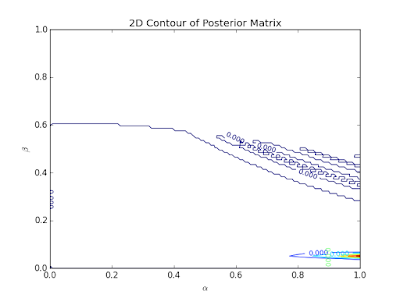Figure 1: Contour plot of Likelihoods for the two parameters.  Colors blue to red represent least to largest Likelihoods respectively.

I know what you're thinking... "This is an ugly plot.  Did something go wrong?"  Well, no.  Everything is fine with my code.  This ugliness insinuates that the priors assigned were far from correct.  Notice how the reddish area is barely visible?  This means that values near 1.0 for $$\alpha$$ yield higher Likelihoods than the blue or colorless regions.  The location of the red area also implies that values near 0.1 seem promising for the $$\beta$$ parameter.Figure 2: Terrible looking cumulative distribution function (CDF) plot.  This CDF was suppose to derive from a normal probability distribution function (PDF).  This plot suggests that the PDF was not of a normal distribution.  This is unfortunate primarily because the parameter and its uncertainties were chosen by assuming that the PDF was Gausssian.

If you want to understand the intricate details about how the plot of Figure 2 came about, read my Grad Level Intro... post and the Grad Level Intro... cont. post.  Those posts will also explain why the PDF and CDF are important.  For now, I'll just say that the purpose of Figure 2 is to provide the best fitting parameter.  There are two reasons why I am not satisfied with the result given by Figure 2.  The first reason for my disdain is the hideosity of the plots (and yes, that is a real word).  The second is the non-Gaussian PDF.  Figure 1 and 2 plots suggest that this fitting did not go well and that the unseen PDF is not of a normal distribution although it should be.Figure 3: Atrocious attempt at finding the best fitting value for $$\beta$$.
The disgusting plots of Figures 2 and 3 only confirm the terrible fitting job that was insinuated first by Figure 1.

Well, all is not lost!  As stated previously, the contour plot gave us a clue as to how we can ameliorate the fitting.  Now, let's run the code with different priors.

>>>
>>>execfile('companion_detect.py')
>>>p_posterior(metal_0, metal_1, alphas=np.linspace(0,0.1,100), betas=np.linspace(1,3,120), plot=True)

Now, our $$\alpha$$ priors range from 0 to 0.1 and our $$\beta$$ priors span from 1 to 3.  Let's check out the results!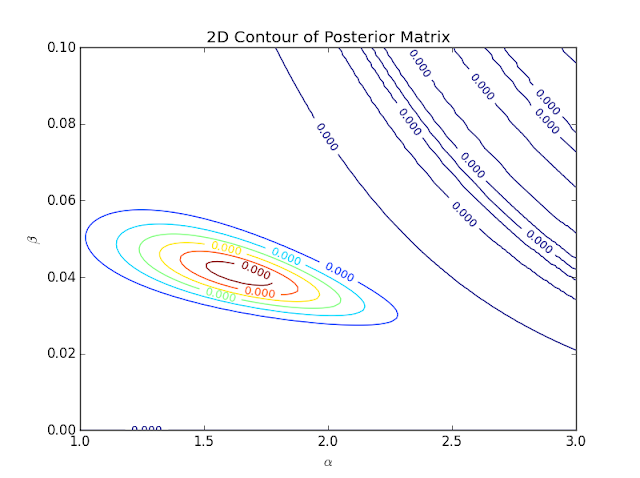Figure 4: Contour plot for the grid search methodology employed.  The Likelihoods calculated are so small that every number shown seems to be the same because only the first 4 digits are shown.  However, I know that the colors represent the different Likelihoods from blue (least) to red (greatest).
That contour plot looks much more aesthetic than the previous one.  However, the number situation can be very confusing at first glance.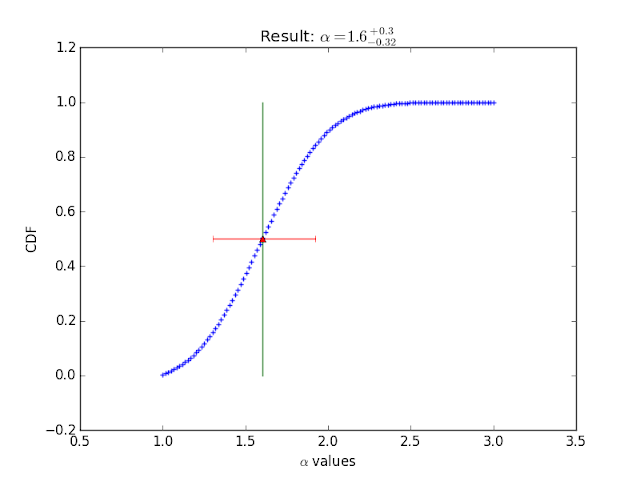Figure 5: Plot of the CDF for the $$\alpha$$ parameter.  The value yielding the best fit was 1.6 for this parameter.Figure 6: Plot of CDF for $$\beta$$ parameter.

There are your results!  Although, I do assume that the results would be more accurate if I were to constrain the range of priors more.

I wanted to show the bad fitting first as a way to convey the detective-like nature of astrophysics researchers.  Researchers, no matter how smart, almost never get the accurate or most satisfactory results on the first try.  This is why one must put on the detective hat and sniff for clues as to what might have gone wrong during the analysis.  I looked for clues in that first contour plot once I noticed how "fishy" it looked.  Figure 1 led me to choosing better priors for my second attempt at the Grid Search.  Moments like that reaffirm my love for plots.  Experiences like that have persuaded me to create many plots as I write complex code.  The graphics can really give a person the ability to see subtle issues that may be visually conspicuous in one's code or methodology that may not be discerned by merely glancing at a bunch of numbers on a computer screen.

## Monday, August 3, 2015

### Astro-Stats & Python : Bayes' Theorem (for Astrophysics)

Back to the Astro-Stats!

In my Bayes' Theorem (for a Coin) post, I introduced Bayes' Theorem for probability along with an example with a Python script.  This time, I will use the same concept for astrophysical data.  Let me first set the stage for this new example and then I'll get to the code so that you can follow along your computer.

My data comprises of the metallicity of stars and the confirmation/denial of a planet detection around a star.  You can see the text file containing this data here.  If you want to follow along, you must copy this information and paste it into your own text file; or, you can download this file using the "wget" command (on that same link) in the Terminal.Figure 1: Snapshot of part of table in the ".txt" file.  The first column is the real name of the star.  The second column is the metallicity of the star relative to the Sun.  This metallicity is the ratio of iron abundance to hydrogen abundance [Fe/H].  Because the Sun is used as the reference, Fe/H for the Sun equals 0.
As with my last analysis with Bayes' Theorem, I must input a prior for each parameter sought, find the Likelihood and then evaluate the new parameters.  In terms of mathematics, the differences between this exercise and the Coin example are conspicuously seen within my Likelihood and Bayes' Probability equations.

The Likelihood is equal to the probability (for the parameters' being measured) of all of the stars with detected planets multiplied by the probability (for the same parameters) of all of the stars without a planet detection.  Because each detection or absence thereof are events that occur independently of each other, the probabilities must be multiplied together to obtain the total probability for the stars with detected planets, as well as those without planets.  This can be described as
$\mathcal{L}=\prod^{k} P(\alpha,\beta) \cdot \prod^{N-k}[ 1-P(\alpha, \beta) ]$
where $$\mathcal{L}$$ is the Likelihood, k is the number of stars with planets, N is the number of stars total, P is the probability of stars with planets, and $$\alpha$$ and $$\beta$$ are parameters.  The rightmost $$\prod$$ term is the total probability of stars without detected planets and the $$\prod$$ term to the left of that is the total probability of stars with detected planets.

Essentially, the Likelihood is being found by multiplying two probabilities.  This corresponds to the generic definition of Likelihood, which can be written as $$\mathcal{L} = \prod^{N}_{i}P_{i}$$  where N is the total number of probabilities.  Likelihood can be defined as the product of all the probabilities.  This was discussed briefly in my Exercise in Likelihood and $$\chi^2$$, continued... post.

For this specific example, the given probability equation is defined as
$P (\alpha, \beta) = \alpha 10^{\beta M}$
where M is the metallicity of one of the observed stars.  Be aware that many statistical applications assume the probability of all outcomes to be equal to each other.  This equation signifies that the probability of each star for having a planet companion is distinct and depends primarily on the metallicity of that star along with the parameters.

Unlike the Coin example, I must now suggest many priors for my parameters $$\alpha$$ and $$\beta$$.  Previously, I provided only 1 prior probability and then let my function use the newfound posterior probability as the subsequent iteration's prior probability.  My methodology is different now because I will use the Grid Search and contour plot strategy to determine which of my priors yielded the highest Likelihoods and thus the highest posterior probabilities.  This method was used in my Grad Level to Intro to Astro-Stats for Undergraduates post and its sequel.  Similar to the equation stated for my Coin example, the posterior Likelihood is described as the following proportion.
$\mathcal{L}_{posterior} \propto \alpha \cdot \beta \cdot \mathcal{L}_{prior}$
Each $$\mathcal{L}_{posterior}$$ will be tracked inside of a matrix, or grid, of numbers that will later be used to create a contour plot.

Now that the math and concepts are (hopefully) clear to you, we can get to the real-life application of all of this knowledge.  My next post will illustrate the results of utilizing this statistical method in my Python script.

to be continued...